# For the following situations, indicate which type of hypothesis test would be used

 Date 20.05.2018 Size 44.76 Kb.

1. For the following situations, indicate which type of hypothesis test would be used.

1. Children who have poor social skills are selected to participate in social skills training. The researcher observes the children on the playground both before and after the social skills training. For each of the 35 children, she records the number of positive peer interactions. She then analyzes the mean before-after difference.

_____ one-sample t-test _____ two independent samples t-test

__X___ matched pairs t-test _____ pooled two sample t-test

1. A researcher randomly assigns 80 high school seniors to either an experimental group where they learn about time management or to a control group where they discuss current events. The researcher then gathers information, for all students, on homework completion. The researcher wants to find out if the time management session is effective for improving homework completion among high school seniors.

_____ one-sample t-test ___X__ two independent samples t-test

_____ matched pairs t-test _____ pooled two sample t-test

1. A researcher want to know if chocolate affects your memory. The researcher find 40 pairs of twins, and randomly selects one twin to eat chocolate and the other twin does not each chocolate. Then all 80 people are given a memory test. The researcher records the score for each person.

_____ one-sample t-test _____ two independent samples t-test

__X___ matched pairs t-test __ ___ pooled two sample t-test

1. A researcher reads that the average time that adults in the United States spend watching TV is 28 hours per week. The researcher randomly selects a sample of 40 college students. The researcher wants to find out if the students also watch TV an average of 28 hours per week.

__X___ one-sample t-test _____ two independent samples t-test

_____ matched pairs t-test _____ pooled two sample t-test

1. College women who are being treated for depression at the campus counseling center were examined for initial depression and then assigned to a drug treatment condition or a placebo control condition. It is assumed the standard deviation is not affected by the drug. At the end of the treatment period, the women take a depression test and their test scores are analyzed to determine if the drug is helpful.

_____ one-sample t-test _____ two independent samples t-test

_____ matched pairs t-test ___X__ pooled two sample t-test

1. A study timed left-handed and right-handed people to see how long it took them to hit a buzzer. The data is shown below:

32 Right handed people had an average of 1.4 seconds and a standard deviation of 0.8 seconds

47 Left handed people had an average of 1.1 seconds and a standard deviation of 0.3 seconds

Test with 10% significance if right handed people are slower than left handed people.

H0: muR≤muL

HA: muR>muL

alpha = 0.10 (given in the problem – they can’t choose differently)

t = (1.4-1.1)/sqrt(.8^2/32+.3^2/47) = 2.0265

0.025

Reject

Our data shows right handed people are slower

1. A study attached microphones to people to count the words they spoke each day.

It is believed the standard deviations for both men and women should be equal.

The average for the 2 men was 6007 words with a standard deviation of 812 words.

The average for the 3 women was 7505 words with a standard deviation of 214 words. Assuming the distribution of words is normal, test whether there is a difference in the number of words spoken between men and women

H0: mu1=mu2

HA: mu1 ne mu2

Alpha=0.05

S=sqrt((1*812^2+2*214^2)/(2+3-2)) = 500

T=(6007-7505)/sqrt(500^2/2+500^2/3) = -3.28

2*0.02

0.04

Reject

Our evidence does show men and women use a different number of words on average

1. They say rock classical music is better for studying than rock music. To investigate the difference a sample of 100 people were chosen to listen to a type of music while taking an IQ test. Half listened to rock music, while the other half listened to classical music. The results are shown below:

Classical Music group: 50 tests, average=104.3, standard deviation=9.4

Rock Music group: 50 tests, average=102.1, standard deviation=7.4

Find a 99% confidence interval for the difference of the two groups on average

(104.3-102.1)+-2.704*sqrt(9.4^2/50+7.4^2/50)=(-2.375, 6.775)

1. A study measured how long men and women stared at the new 144” HD HGTV. Of the 47 men the average was 12.4 minutes with a standard deviation of 5.3 minutes. Of the 27 women the average was 6.4 minutes with a standard deviation of 1.2 minutes. The average difference was 6 minutes and the standard deviation of the differences was 0.68. Find a 92% confidence interval for the average difference between the two genders.

Cannot do this problem – there are not enough women sampled

1. The distribution of wait times for a movie to load on Hulu is normally distributed with a standard deviation of 2.4 seconds. What changes the mean is how long it takes Windows to give the Hulu player priority in the process queue. I want to know the average on my old computer, so I randomly sample it 4 times, and I get an average of 480 seconds. Find a 96% confidence interval for the average time my old computer takes to play a movie on Hulu.

t=3.482 (475.82, 484.18)

1. Michael is planning to advertise by putting magnetic ads on cars, and he has an option of putting the ads on white cars or red cars. To find out he convinces his friends to randomly paint their cars either red or white (the order of which color was used first was chosen randomly). Each car is parked in the Taco Bell parking lot and Michael notes how many people stop to check out the car. Then the next day the car is painted the different color, and parked in the same spot.

Michael assumes that the number of people who check out an ad on a car is normally distributed, and he collected the data below. Show all 7 steps for the hypothesis test to determine if the color of a car has an effect on the number of people who look at the car.

 Red White Justin’s Car 7 10 Kenneth’s Car 5 8 Ryan’s Car 8 7 Omer’s Car 7 12 Sarah’s Car 12 11 Shawn’s Car 10 16

Variance of cars when painted red: 6.17 people2

Variance of cars when painted white: 10.27 people2

Unpooled sum of variances: 16.44 people2

Pooled variance of cars: 8.22 people2

Matched pairs variance: 8.70 people2

Variational Delta: 4.1 people2

First it is important to notice that this is a matched pairs t-test.

The data is paired by each individual’s car. This is not ANOVA because pairs of data are linked, not independent. This is not regression because the colors red and white are not numerical. This is not a chi-squared goodness of fit test because we are not testing whether Michael’s friends tend to have red cars or white cars. In fact the only reason we care about who’s car it was is to emphasize that the data is paired along each row.
The mean is -2.5 (red-white), variance 8.7, sample size is 6 (that’s all we need)

H­­: μredwhite

H­­: μred≠μwhite

α=0.05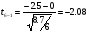0.025Double because this is a two-tailed test

0.05

Since p-value>.05, Fail to reject

Conclude that there is not enough evidence to say that the color of the car affects the number of people who look at the car.

1. An engineer believes the average weight of a tree is 6000 pounds. He feels it’s safe to assume the weights are normally distributed. He weighs three randomly selected trees and records their weight:

6000 pounds

13000 pounds

51500 pounds
Test whether the engineer is correct.
H0:mu=6000

HA:mu ne 6000

Alpha = 0.05 (or something reasonable)

Xbar = 23500

Sd=sqrt((6000-23500)^2+(13000-23500)^2+(51500-23500)^2)/(3-1))

Sd = 24500

Df=2

T=(23500-6000)/(24500/sqrt(3))=1.24

2*.15< p-value <2*0.2

.3 < p-value < .4

Fail to Reject

Our data does not show the rancher is incorrect

1. An investigator wishes to determine whether alcohol consumption causes deterioration in the performance of automobile drivers. Before the driving test, subjects drank a glass of orange juice which, in the case of the treatment group, is laced with two ounces of vodka. Performance is measured by the number of errors made on a driving simulator. 120 volunteer subjects are randomly assigned, in equal numbers, to the two groups. It is believed that the variance in both groups will be the same. For subjects in the treatment group, the mean number of errors (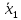) equals 26.4 and the standard deviation (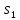) equals 13.99. For subjects in the control group, the mean number of errors (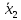) equals 18.6, and the standard deviation (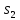) equals 12.15. Find a 95% confidence interval for the difference in errors for the alcohol group compared to the control group.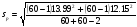=13.1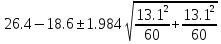=(3.05, 12.55)

1. A database manager wants to know if plugging in a speaker slows down a computer. She randomly selects 7 different computer companies and gets two computers from each company. For each company she has one computer where speakers are plugged in, and one where they are not. Then all 14 computers calculate the ten billionth digit of pi. Assume computer times are normally distributed and that the speakers do not affect the standard deviation. Given the data below calculate a 99.8% confidence interval for the difference in average time.

Computers without speakers

Computers: 7

Mean time: 850 minutes

Standard deviation: 25 minutes

Computers with speakers

Computers: 7

Mean time: 910 minutes

Standard deviation: 45 minutes

The standard deviation of the differences: 21 minutes

Pooled Standard deviation: 36.4 minutes

THIS one is the matched pairs example (each pair is of the same company). Also a t=5.208

(-101.27, -18.66)

1. 40 volunteers were selected to take part in a “Twinkie diet” experiment. The subjects were weighed first. Of the two who were the lightest weight one was chosen to be control and the other “Twinkie”. Then of the next two highest weights one was control, the other “Twinkie”. This pattern continued until half were in the Twinkie group and the other half were the control group. The data is shown below.

Twinkie group:

The twenty people averaged 40 pounds gained with a standard deviation of 22 pounds
Control group:

The twenty people averaged 8 pounds gained with a standard deviation of 10 pounds

Matched pairs standard deviation: 6 pounds

Pooled standard deviation: 17 pounds

Find a 90% confidence interval for the difference in average pounds gained.
Not a large enough sample size to do